# NCERT Solutions for Class 7 Maths Chapter 13 Exponents and Powers Ex 13.1

NCERT Solutions for Class 7 Maths Chapter 13 Exponents and Powers Ex 13.1 are part of NCERT Solutions for Class 7 Maths. Here we have given NCERT Solutions for Class 7 Maths Chapter 13 Exponents and Powers Ex 13.1.

 Board CBSE Textbook NCERT Class Class 7 Subject Maths Chapter Chapter 13 Chapter Name Exponents and Powers Exercise Ex 13.1 Number of Questions Solved 8 Category NCERT Solutions

## NCERT Solutions for Class 7 Maths Chapter 13 Exponents and Powers Ex 13.1

Question 1.
Find the value of:

1. 26
2. 93
3. 112
4. 54.

Solution:

1. 26 = 2 × 2 × 2 × 2 × 2 × 2 = 64
2. 93 = 9 × 9 × 9 = 729
3. 112 = 11 × 11 = 121
4. 54 = 5 × 5 × 5 × 5 = 625.

Question 2.
Express the following in exponential form:

1. 6 × 6 × 6 × 6
2. t × t
3. b × b × b × b
4. 5 × 5 × 7 × 7 × 7
5. 2 × 2 × a × a
6. a × a × a × c × c × c × c × d.

Solution:

1. 6 × 6 × 6 × 6 = 64
2. t × t = t2
3. b × b × b × b = b4
4. 5 × 5 × 7 × 7 × 7 = 52 × 73
5. 2 × 2 × a × a = 22 × a2
6. a × a × a × c × c × c × c × d = a3 × c4 × d.

Question 3.
Express each of the following numbers using the exponential notation:
(i) 512
(ii) 343
(iii) 729
(iv) 3125.
Solution: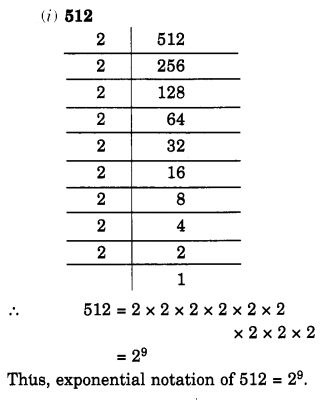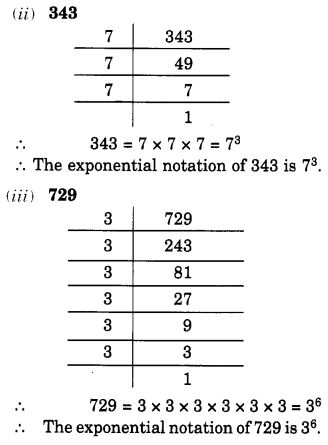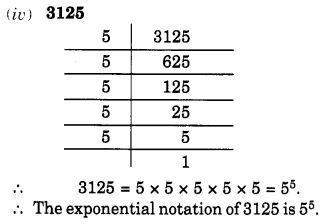Question 4.

Identify wherever possible, in each of the following?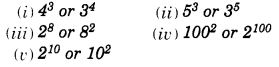Solution: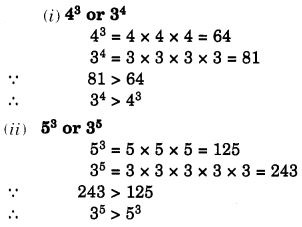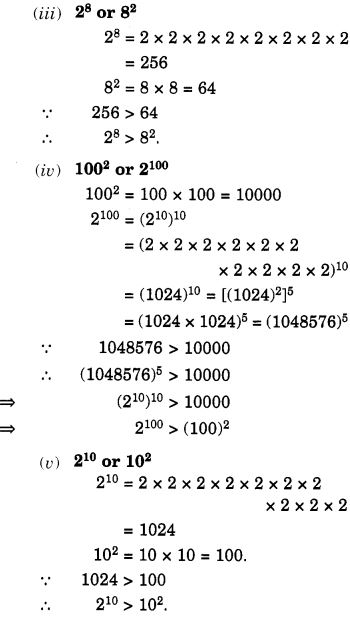Question 5.
Express each of the following as a product of powers of their prime factors:
(i) 648
(ii) 405
(iii) 540
(iv) 3600
Solution: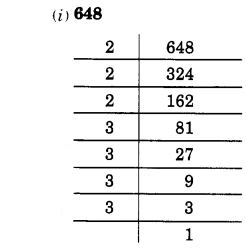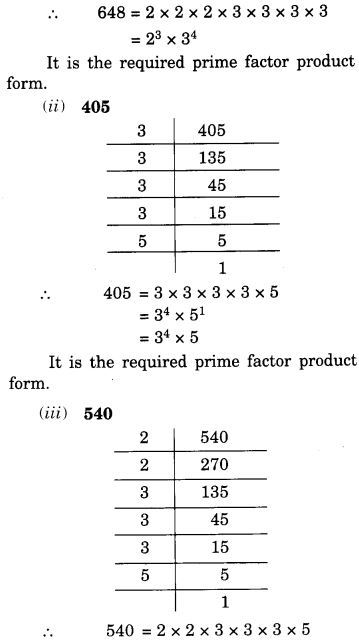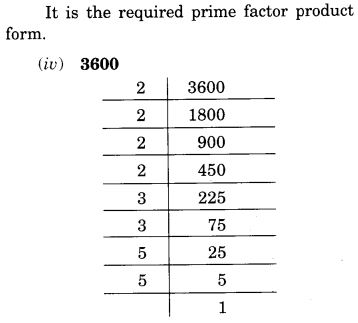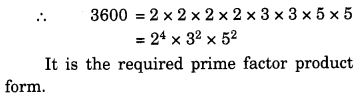Question 6.
Simplify: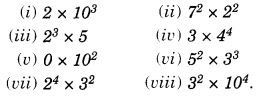Solution: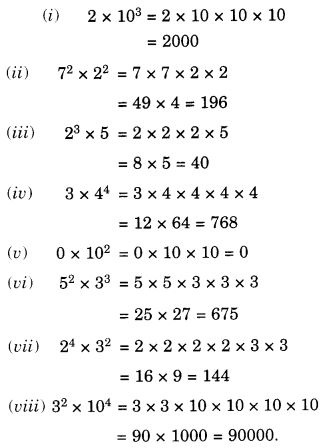Question 7.
Simplify: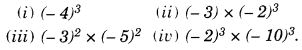Solution: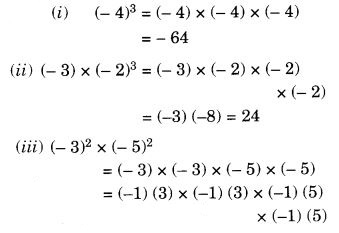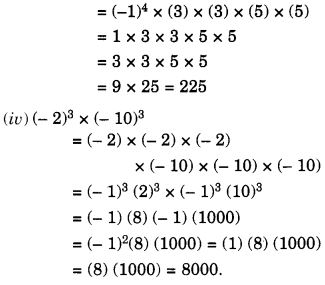Question 8.
Compare the following numbers: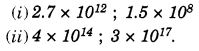Solution: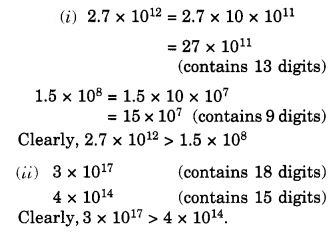We hope the NCERT Solutions for Class 7 Maths Chapter 13 Exponents and Powers Ex 13.1 help you. If you have any query regarding NCERT Solutions for Class 7 Maths Chapter 13 Exponents and Powers Ex 13.1, drop a comment below and we will get back to you at the earliest.